## Example Questions

### Example Question #1 : Fractions And Percentage

Luke went to the grocery store. It took him 15 minutes to drive to the store from his house, 30 minutes to shop, 5 minutes to put groceries in his car, and 20 minutes to drive home due to traffic.

What percentage of the trip did Luke spend inside his car?

45%

75%

50%

15%

35%

50%

Explanation:

You take 15 + 20 = 35 minutes as total time spent in the car.

Then, you take 15 + 20 + 5 + 30 = 70 minutes as total trip time.

We take (35/70) x 100% = 50%

### Example Question #1 : Percentage

There are 300 sandwiches at a company-wide picnic. If half of the sandwiches are tuna, and the provider mistakenly uses expired tuna in three-fifths of those sandwiches, what percentage of total sandwiches still remain edible?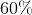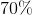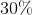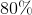Explanation:

This multi-part question makes use of both fractions and percentages, as well as some tricky language if you are not paying close attention.

First off, determine what three-fifths of the 150 tuna sandwiches is: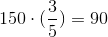Now, refer back to the question, which asks how many TOTAL sandwiches (tuna and non-tuna) still remain edible. This is simple arithmetic.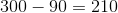Finally, find what percentage this equals: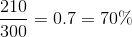### Example Question #2 : Fractions And Percentage

Express the fraction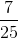as a percentage.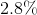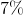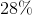Explanation:

Converting a fraction to a percentage can be done in two ways.  The first way involves recalling that a percent is just a fraction of 100 (per cent).  Therefore, the percent equivalent tois the numerator of the equivalent fraction whose denominator is 100.  In order to convertto a fraction over 100, I need to multiply the numerator and denominator each by 4.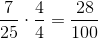The other approach is to convert the fraction to a decimal first by dividing 7 by 25.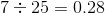To convert a decimal to a percent, just shift the decimal point two places to the right.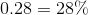With this method, our answer is again 28%.

### Example Question #10 : Percentage

Convert to a percentage: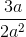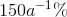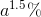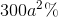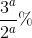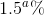Explanation:

Despite the presence of a variable, the rule remains the same: To convert a fraction to a percentage, multiply the numerator by 100 and solve the fraction.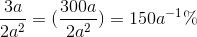### Example Question #1 : How To Find Percentage From A Fraction

A politician promises to spend a minimum of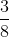of his budget on improving roads. What is the minimum percent of the budget that must be spent on roads to keep this promise?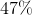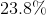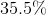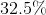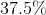Explanation:

To find a percentage from a fraction, multiply the numerator by, then solve the fraction.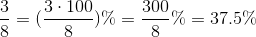Thus, the politician must spend at leastof the budget on roads.

### Example Question #1 : How To Find Percentage From A Fraction

Convert to a percentage: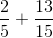Round to the nearest integer.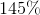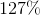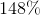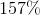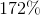Explanation:

We can solve this problem either by adding the two fractions and then converting to a percentage, or by converting each fraction to a percentage before adding them. We'll use the first method in this case: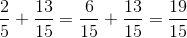Now, just as before, multiply our numerator byand solve the fraction: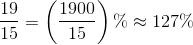### All ACT Math Resources